S t a r t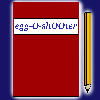Diary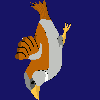Download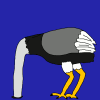Insight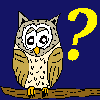Questions?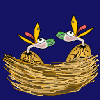Food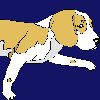Links I m p r i n tKlick hier für die deutschsprachige Version.

 <<<     Range events     >>> At this column you can learn to create your own egg-O-shOOter. How to direct objects was described at the previous chapter. Now you will learn how to trigger range events. Objects can be deactivated or activated as soon as this range event appears. Try to let an egg fall from the ceiling as soon as the fly reached it. Therefor we need an egg and a fly: #1# ObjectNumber=1       #1#Fliege / Fly ObjectFront=1 ObjectStartActive=1 ObjectTexture=11/70,12/30 ObjectF=R2 ObjectD=-1 ObjectSecMin=-1 ObjectSecMax=-1 ObjectConnect=-1 ObjectKoordPre=-1 ObjectHitDirection=1 ObjectXMin=-100 ObjectXMax=-100 ObjectYMin=-160 ObjectYMax=-160 ObjectDMin=20 ObjectDMax=20 ObjectAMin=0 ObjectAMax=0 ObjectVXMin=-100 ObjectVXMax=-100 ObjectVYMin=+20 ObjectVYMax=+50 ObjectVRotMin=0 ObjectVRotMax=0 ObjectRotAxis=3 ObjectVGrowMin=0 ObjectVGrowMax=0 ObjectGravMin=0 ObjectGravMax=0 ObjectMoveXMin=-800 ObjectMoveXMax=+800 ObjectMoveYMin=+212 ObjectMoveYMax=+220 ObjectMoveAMin=-360 ObjectMoveAMax=+360 ObjectMoveRMin=1 ObjectMoveRMax=1000 ObjectMoveType=11 ObjectType=1 ObjectBenefitMin=*0,*0,*0,*0,*0,*0,*0,*0,*0,*0,*0,*0,*0 ObjectBenefitMax=*0,*0,*0,*0,*0,*0,*0,*0,*0,*0,*0,*0,*0 ObjectMirror=0 ObjectMovePointing=1 ObjectHitSound= ObjectAppearSound= ObjectHitTextEnglish= ObjectHitTextGerman= #1# ObjectNumber=2       #1#Ei von Decke fallend / Egg falling from ceiling ObjectFront=1 ObjectStartActive=0 ObjectTexture=90 ObjectF=-1 ObjectD=H2 ObjectSecMin=-1 ObjectSecMax=-1 ObjectConnect=-1 ObjectKoordPre=1 ObjectHitDirection=1 ObjectXMin_0 ObjectXMax_0 ObjectYMin_0 ObjectYMax_0 ObjectDMin=20 ObjectDMax=20 ObjectAMin=0 ObjectAMax=0 ObjectVXMin=0 ObjectVXMax=0 ObjectVYMin=+20 ObjectVYMax=+20 ObjectVRotMin=50 ObjectVRotMax=100 ObjectRotAxis=3 ObjectVGrowMin=0 ObjectVGrowMax=0 ObjectGravMin=+10 ObjectGravMax=+10 ObjectMoveXMin=-800 ObjectMoveXMax=+800 ObjectMoveYMin=-200 ObjectMoveYMax=+200 ObjectMoveAMin=-360 ObjectMoveAMax=+360 ObjectMoveRMin=1 ObjectMoveRMax=1000 ObjectMoveType=3 ObjectType=3 ObjectBenefitMin=*0,*0,*0,*0,*0,*0,*0,*0,*0,*0,*0,*0,*0 ObjectBenefitMax=*0,*0,*0,*0,*0,*0,*0,*0,*0,*0,*0,*0,*0 ObjectMirror=0 ObjectMovePointing=0 ObjectHitSound= ObjectAppearSound= ObjectHitTextEnglish= ObjectHitTextGerman= Save 'themes.txt', press 'N'-key and direct the fly to the ceiling. The egg appears really at the point of the ceiling where at the fly reached it. The egg falls, forced by gravity, down to the bottom frame and bounces at the edge of the playground. When being hit, the egg bursts and is deactivated. So far so good, but where is the fly? We did not deactivate it when reaching the ceiling! Well, as soon as the fly leaves the defined area, the range event is triggered and as wanted the egg is activated. Therewith the fly would still be outside the area and trigger the range event once more! To avoid this accident, the object triggering the range event is automatically deactivated by the egg-O-shOOter. If the fly should stay at the scene, we have to define a subsequent fly. So we need three objects to realize this example: #1# ObjectNumber=3       #1#Nachfolge-Fliege / Subsequent fly ObjectFront=1 ObjectStartActive=0 ObjectTexture=12 ObjectF=-1 ObjectD=-1 ObjectSecMin=-1 ObjectSecMax=-1 ObjectConnect=-1 ObjectKoordPre=1 ObjectHitDirection=-1 ObjectXMin_0 ObjectXMax_0 ObjectYMin_0 ObjectYMax_0 ObjectDMin=20 ObjectDMax=20 ObjectAMin=+180 ObjectAMax=+180 ObjectVXMin=0 ObjectVXMax=0 ObjectVYMin=0 ObjectVYMax=0 ObjectVRotMin=0 ObjectVRotMax=0 ObjectRotAxis=3 ObjectVGrowMin=0 ObjectVGrowMax=0 ObjectGravMin=0 ObjectGravMax=0 ObjectMoveXMin=-800 ObjectMoveXMax=+800 ObjectMoveYMin=-200 ObjectMoveYMax=+220 ObjectMoveAMin=-360 ObjectMoveAMax=+360 ObjectMoveRMin=1 ObjectMoveRMax=1000 ObjectMoveType=3 ObjectType=1 ObjectBenefitMin=*0,*0,*0,*0,*0,*0,*0,*0,*0,*0,*0,*0,*0 ObjectBenefitMax=*0,*0,*0,*0,*0,*0,*0,*0,*0,*0,*0,*0,*0 ObjectMirror=0 ObjectMovePointing=1 ObjectHitSound= ObjectAppearSound= ObjectHitTextEnglish= ObjectHitTextGerman= If you want the fly to hang around with it's feet at the ceiling, you have to set ObjectAMin/Max = 180 (at object number 3!). ObjectF at object 1 (!) has to be set to ObjectF=R2,R3 to activate the subsequent fly besides the egg. Start the level and look if the egg appears as predicted and if the fly stays at the ceiling.It works? all right! The further values for ObjectMoveType should be no more secret for you. While ObjectMoveType=7 causes the range event if the fly reaches the area fortuitous, you can't dissuade the fly from reaching the area if ObjectMoveType is set to 6. The fly is guided to the area. Set ObjectMoveType=4 and the range event is triggered as soon as the fly leaves the area. Note that the fly should not be outside the target area at the point it is activated. Elsewise the fly is immediately deactivated and you won't see it. ObjectMoveType=11 is similar to the value 7, but the fly won't disappear at the edge of the playground.